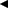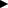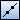# Creating Dividing Point

Create a point at the position where 2 points or specified shapes are divided.
The following 3 methods can be used to create a dividing point.

• Specify the divided number.
• Specify the dividing length.
• Specify the dividing angle.

To display a command parameter to be executed, click/of command parameters, or press Tab key or / key on the keyboard.

1. Point [Point] on [Shape] menu, and click [Dividing Point].
Then you will be able create a dividing point.
2.  Supplemental ▪ You can enter into the dividing point creating status by clickingon [Shape] toolbar.

### When specifying the start and end points

1. Click the start point of the dividing point on the drawing.
2. Click the end point of the dividing point on the drawing.
3.  Supplemental ▪ You can specify the start and end points by inputting X and Y coordinates in [Start Point] and [End Point] boxes on Command Window.
When specifying the divided number
1. Input a divided number between 2 points in [Divided Number] box on Command Window.
The length between 2 points will be equally divided into the number specified, and points will be created in individual dividing points.
When specifying the dividing length
1. Input a dividing length between 2 points in [Dividing Length] box on Command Window.
The length between 2 points will be divided in a specified length, and points will be created in individual dividing points.

### When specifying the shape

1. Click a shape to become a base point for creating a dividing point.

2. When specifying the divided number
1. Input a divided number in [Divided Number] box on Command Window.
Shapes will be equally divided into the number specified, and points will be created in individual dividing points.
When specifying the dividing length
1. Input a dividing length in [Dividing Length] box on Command Window.
Shapes will be divided in a specified length, and points will be created in individual dividing points.
If a shape selected in Step 2 is other than a circle and ellipse, the end point nearer to the clicked position in the shape will be a start point of dividing.
When specifying the dividing angle (when dividing a circle, arc, ellipse or elliptical arc)
1. Input a dividing angle in [Dividing Angle] box on Command Window.
Shapes will be divided in a specified angle, and points will be created in individual dividing points.
2. Specify the start point for dividing when a circle or ellipse is selected in Step 2.
When specifying the start angle for dividing
Switch the parameter on Command Window to [Start Angle] box, and input the start angle for dividing.
When specifying the start point for dividing
Switch the parameter on Command Window to [Start Point] box, and click the start point for dividing on the drawing.
3.  Supplemental ▪ You can specify the start point by inputting X and Y coordinates in [Start Point] box on Command Window.